# Disc

The circumference of the disk is 78.5 cm. What is the circumference of the circular arc of 32° on the disc?

o =  31.9651 cm

### Step-by-step explanation: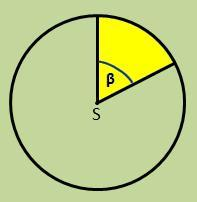Did you find an error or inaccuracy? Feel free to write us. Thank you!#### You need to know the following knowledge to solve this word math problem:

We encourage you to watch this tutorial video on this math problem:

## Related math problems and questions:

• Arc-sectorarc length = 17 cm area of sector = 55 cm2 arc angle = ? the radius of the sector = ?
• Circle sectorThe circular sector with a central angle 160° has an area 452 cm2. Calculate its radius r.
• ArcCircle arc corresponding to angle is 32° is 28 dm long. What is the length of the entire circle?
• Arc and segmentCalculate the length of circular arc l, area of the circular arc S1 and area of circular segment S2. Radius of the circle is 11 and corresponding angle is (2)/(12) π.
• Cone A2VThe surface of the cone in the plane is a circular arc with central angle of 126° and area 415 cm2. Calculate the volume of a cone.
• Circular arcCalculate the length of the circular arc if the diameter d = 20cm and the angle alpha = 142 °
• Length of the arcWhat is the length of the arc of a circle k (S, r=68mm), which belongs to a central angle of 78°?
• Arc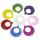What area of a circle occupied the flowers planted in the arc of a circle with radius 3 m with central angle 45°?
• SectorThe perimeter of a circular sector with an angle 1.8 rad is 64 cm. Determine the radius of the circle from which the sector comes.
• Quarter of a circleCalculate the circumference of a quarter circle if its content is S = 314 cm2.
• ParallelogramFind the parallelogram's perimeter, where base a = 8 cm, height v = 3 cm, and angle alpha = 35° is the magnitude of the angle at vertex A.
• Quarter circleWhat is the radius of a circle inscribed in the quarter circle with a radius of 100 cm?
• Circle sectionEquilateral triangle with side 33 is inscribed circle section whose center is in one of the vertices of the triangle and the arc touches the opposite side. Calculate: a) the length of the arc b) the ratio betewwn the circumference to the circle sector and
• The length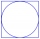The length of the circle is 24 cm. What is the length of the circular arc of the corresponding angle 30°?
• Circle arcCalculate the circular arc area in m2 where the diameter is 263 dm, and a central angle is 40°. Result round to three decimal places.
• Track arc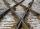Two straight tracks is in an angle 74°. They will join with circular arc with radius r=1127 m. How long will be arc connecting these lines (L)? How far is the center point of arc from track crossings (x)?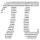Convert 270° to radians. Write result as multiple of number π.